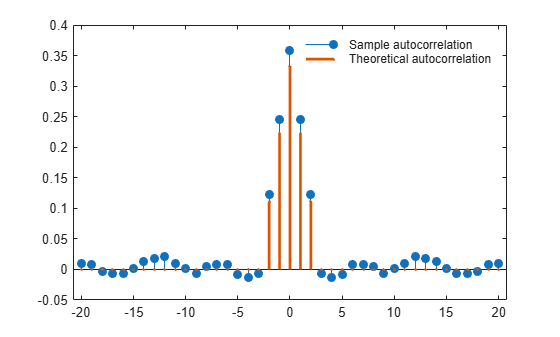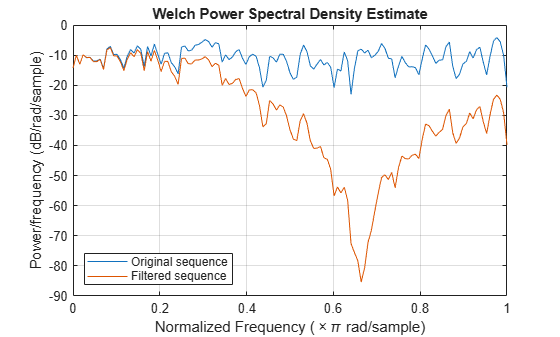Main Content

# Autocorrelation of Moving Average Process

This example shows how to introduce autocorrelation into a white noise process by filtering. When we introduce autocorrelation into a random signal, we manipulate its frequency content. A moving average filter attenuates the high-frequency components of the signal, effectively smoothing it.

Create the impulse response for a 3-point moving average filter. Filter an N(0,1) white noise sequence with the filter. Set the random number generator to the default settings for reproducible results.

h = 1/3*ones(3,1); rng default x = randn(1000,1); y = filter(h,1,x);

Obtain the biased sample autocorrelation out to 20 lags. Plot the sample autocorrelation along with the theoretical autocorrelation.

[xc,lags] = xcorr(y,20,'biased'); Xc = zeros(size(xc)); Xc(19:23) = [1 2 3 2 1]/9*var(x); stem(lags,xc,'filled') hold on stem(lags,Xc,'.','linewidth',2) lg = legend('Sample autocorrelation','Theoretical autocorrelation'); lg.Location = 'NorthEast'; lg.Box = 'off';The sample autocorrelation captures the general form of the theoretical autocorrelation, even though the two sequences do not agree in detail.

In this case, it is clear that the filter has introduced significant autocorrelation only over lags [-2,2]. The absolute value of the sequence decays quickly to zero outside of that range.

To see that the frequency content has been affected, plot Welch estimates of the power spectral densities of the original and filtered signals.

[pxx,wx] = pwelch(x); [pyy,wy] = pwelch(y); figure plot(wx/pi,20*log10(pxx),wy/pi,20*log10(pyy)) lg = legend('Original sequence','Filtered sequence'); lg.Location = 'SouthWest'; xlabel('Normalized Frequency (\times\pi rad/sample)') ylabel('Power/frequency (dB/rad/sample)') title('Welch Power Spectral Density Estimate') gridThe white noise has been "colored" by the moving average filter.

## Support

#### Deep Learning for Signal Processing with MATLAB

Download white paper